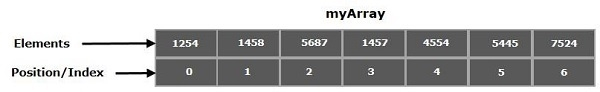# What are the different ways to iterate over an array in Java?

In general, arrays are the containers that store multiple variables of the same datatype. These are of fixed size and the size is determined at the time of creation. Each element in an array is positioned by a number starting from 0.You can access the elements of an array using name and position as −

System.out.println(myArray);
//Which is 1457

## Creating an array in Java

In Java, arrays are treated as referenced types you can create an array using the new keyword similar to objects and populate it using the indices as −

int myArray[] = new int;
myArray = 1254;
myArray = 1458;
myArray = 5687;
myArray = 1457;
myArray = 4554;
myArray = 5445;
myArray = 7524;

Or, you can directly assign values with in flower braces separating them with commas (,) as −

int myArray = { 1254, 1458, 5687, 1457, 4554, 5445, 7524};

## Iterating over an array

You can iterate over an array using for loop or forEach loop.

Using the for loop − Instead on printing element by element, you can iterate the index using for loop starting from 0 to length of the array (ArrayName.length) and access elements at each index.

## Example

public class IteratingArray {
public static void main(String args[]) {
//Creating an array
int myArray[] = new int;
//Populating the array
myArray = 1254;
myArray = 1458;
myArray = 5687;
myArray = 1457;
myArray = 4554;
myArray = 5445;
myArray = 7524;
//Printing Contents using for loop
System.out.println("Contents of the array: ");
for(int i=0; i<myArray.length; i++) {
System.out.println(myArray[i]);
}
}
}

## Output

Contents of the array:
1254
1458
5687
1457
4554
5445
7524

Using the for each loop − Since JDK 1.5, Java introduced a new for loop known as foreach loop or enhanced for loop, which enables you to traverse the complete array sequentially without using an index variable.

You can iterate the contents of an array with less effort using this.

## Example

import java.util.Arrays;
public class IteratingArray {
public static void main(String args[]) {
//Creating an array
int myArray[] = new int;
//Populating the array
myArray = 1254;
myArray = 1458;
myArray = 5687;
myArray = 1457;
myArray = 4554;
myArray = 5445;
myArray = 7524;
//Printing Contents using for each loop
System.out.println("Contents of the array: ");
for (int element: myArray) {
System.out.println(element);
}
}
}

## Output

Contents of the array:
[1254, 1458, 5687, 1457, 4554, 5445, 7524]